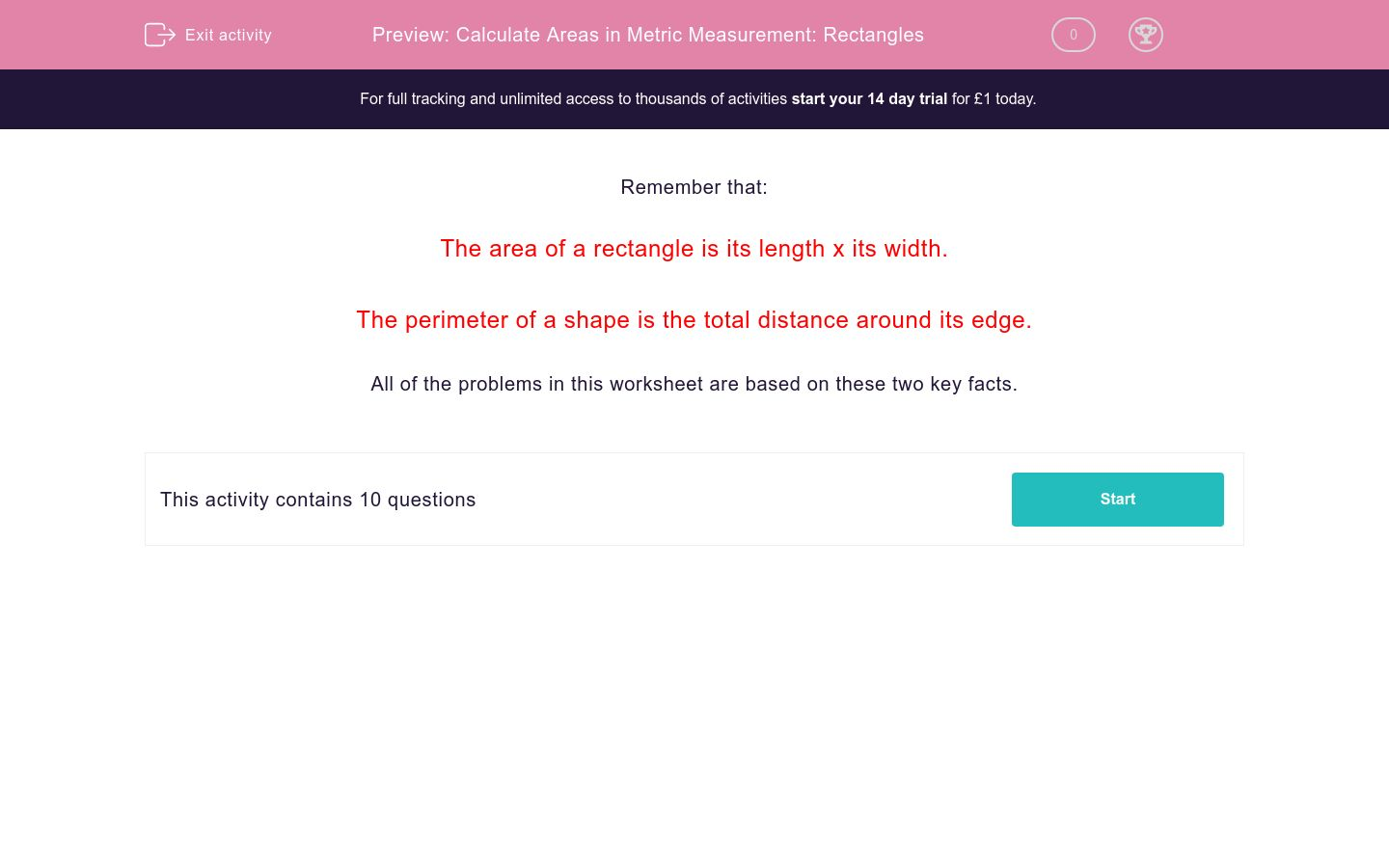# Calculate Areas in Metric Measurement: Rectangles

In this worksheet, students calculate the area of rectangles using metric measurements.Key stage:  KS 2

Curriculum topic:   Maths and Numerical Reasoning

Curriculum subtopic:   Perimeter and Area

Difficulty level:#### Worksheet Overview

Remember that:

The area of a rectangle is its length x its width.

The perimeter of a shape is the total distance around its edge.

All of the problems in this worksheet are based on these two key facts.

### What is EdPlace?

We're your National Curriculum aligned online education content provider helping each child succeed in English, maths and science from year 1 to GCSE. With an EdPlace account you’ll be able to track and measure progress, helping each child achieve their best. We build confidence and attainment by personalising each child’s learning at a level that suits them.

Get started